< 体育竞技 < 实况足球2010# 实况足球2010

• 截图
• 相关
• 评论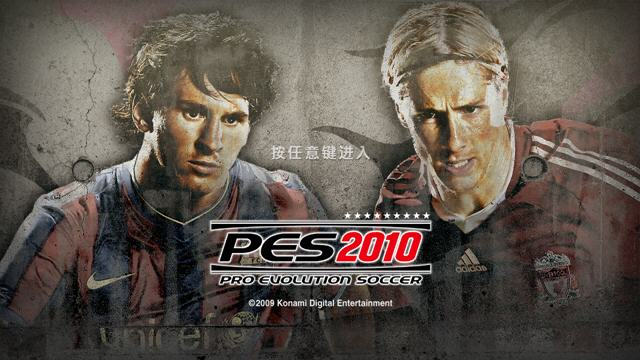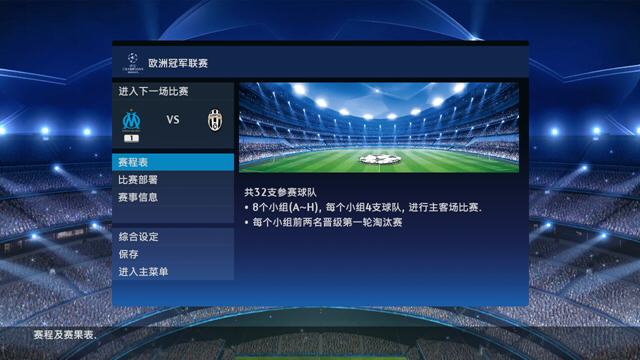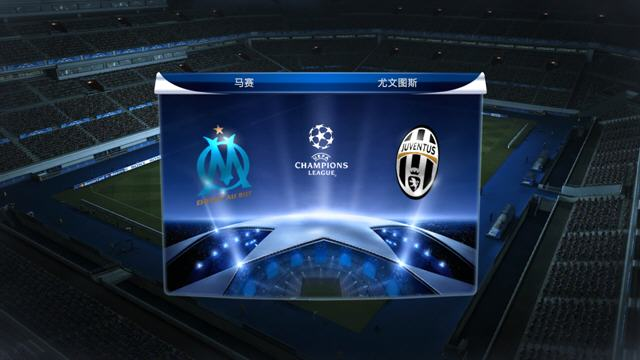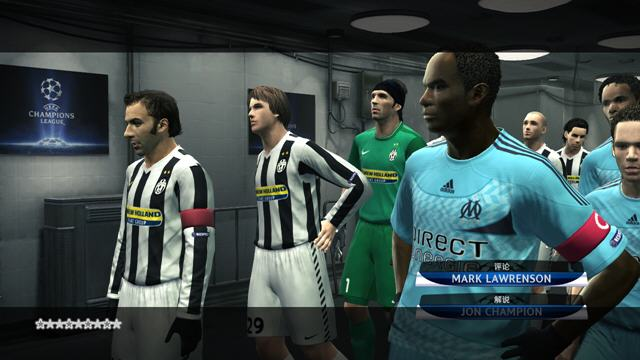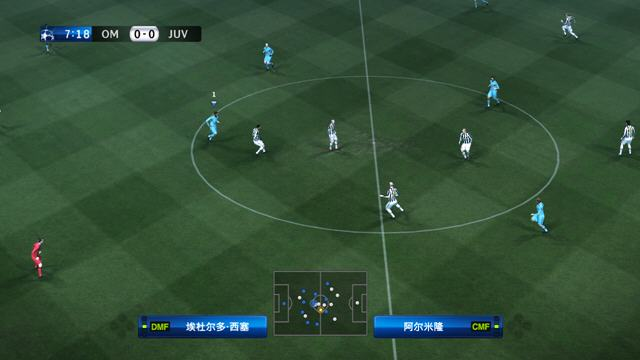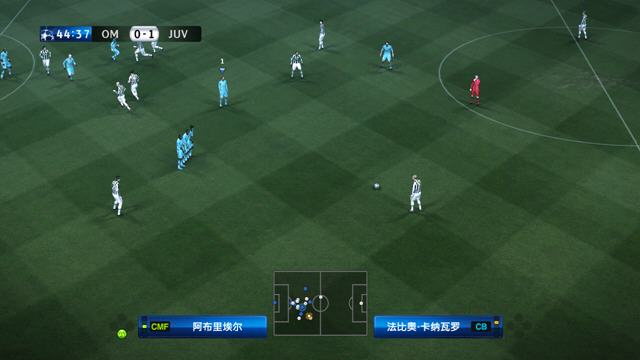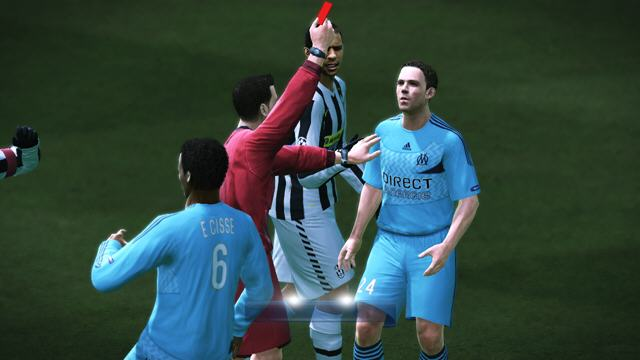#### 实况足球2010简介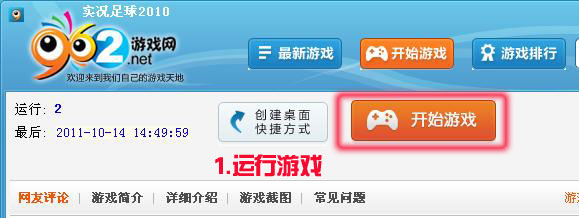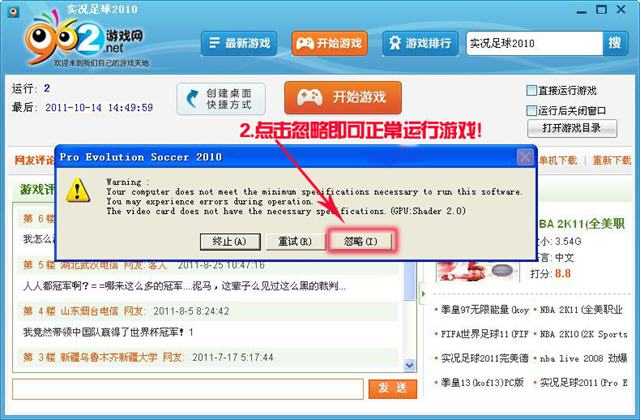《实况足球2010》最新转会存档 最新4.0版(更新至冬季转会)：http://www.962.net//patch/5710.html

《实况足球2010》是由科乐美公司于2009年推出的PES系列最新作品，在2010作品中主打对抗赛，为了让玩家们能体验到真是足球带来的对抗精彩内容，无论是画面上还是动作细节上都非常出色。

### 【游戏介绍】

konami宣布新作《实况足球2010》将在很多方面有重大改进，而且这些改进方案是在广泛听取FANS的反馈意见并加以详细分析之后得出的。Konami官方把《实况足球2010》描述成“史上最逼真的足球模拟游戏”，其对应平台为PS3、X360、PSP、PS2和Wi

### 【游戏操作】

Q=切换光标=L1
E=加速=R1
W=前插和门将出击=△
A=长传和铲断=O
X=短传和抢球=X
D=射门和解围=口
Z=切换战术键 =L2
C=调整弧线和假动作=R2
Q+E=强行加速突破=R1+L1
E+C=强制取消一切动作=R1+R2
Q+W=过顶前插= R1+△
Q+X=2过1=L1+X
Q+D=吊射=L1+口
E+D=挑射=R1+口
Q+ASDX=改变整体战术=R1+口△OX

W直塞
X短传
A射门
D长传 铲球
Q L1 换人
E L2 加速 &踩单车（大）
Z R1
C R2 踩单车 （小）

### 【疑难问题】

《实况足球2010》画面过亮解决方法：